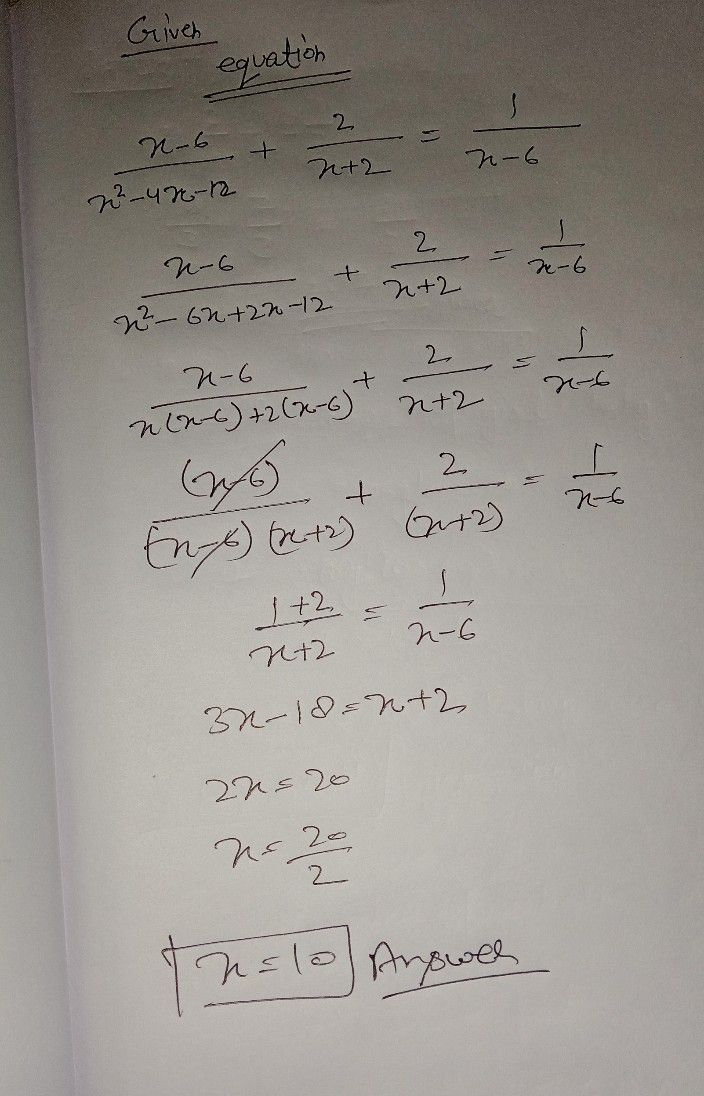Symbol
Problem3. Apply the Distributive Property and then simplify. 4. Find all the $possiblc$ values of x $x=5$ 5. Check each value by substituting into the original equation and reject any extraneous $0ot/s$ 2. Rational Equation $\dfrac {x-6} {x^{2}-4x-12}+\dfrac {2} {x+2}=\dfrac {1} {x-5}$ 1. Find the Least Common Denominator $LcD\right)$ 2. Multiply both sides of the equation by its the $LCD$ 3. Apply the Distributive Property and then simplify. 4. Find all the $possible$ values of x. $x=10$ 5. Check each value by substituting into original equation and reject any extraneous $oot/s$ 3. Rational Inequality $-$ $\dfrac {2\left(x-4\right)} {x}<-4$ 1. Put the rational inequality in $gen∈\pi 2$ form. $\dfrac {R\left(x\right)} {Q\left(x\right)}>0$ $e>$ can $beTeplaCed$ $by<\leq$ where and 2 $2.$ $1Vnte$ the inequality $int0$ a single rational expression on the left side. 17
10th-13th grade
Algebra
Search count: 109
SolutionQanda teacher - henryseeStudent
Thank you maaam/sir